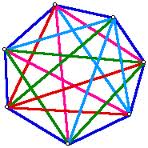## Looking for something to do with triangles?

I was scratching around for some things to do with triangles to show the kids some interesting properties of these cool shapes. I didn't want to get into Pythagoras at this point but lots of the websites and Youtube clips I found were pretty pedestrian.

So, here’s my contributions about what you can do with triangles. Hopefully they are a bit more stimulating and provocative than “put a triangle on top of a square to make a house”…

## 1. How many degrees in the sum of the angles of a triangle?

Cut out a triangle. Put a dot in each corner. Cut off the corners. Reassemble them to make a straight line.
How many degrees are there in the sum of the angles of a triangle?
What would happen if you used the corners from a quadrilateral? pentagon? dodecagon?

## 2. Diagonals making new triangles

Divide polygons up into triangles using diagonals going from one corner to another. How many triangles do you end up with if you start with:
a) a quadrilateral and join up each corner with straight line diagonals?
b) a pentagon and join up each corner with straight line diagonals?
c) a hexagon and join up each corner with straight line diagonals?What is the pattern you can see emerging here? How many triangles would you expect to make with an octagon? And is there something special about the middle shape you create when you start with an odd-sided polygon?

## 3. Cross sections of a tetrahedron

Make a playdough model of a tetrahedron. Can you make a shadow that is:
a) an equilateral triangle?
b) a square

Use a plastic knife to cut a cross section. Can you make a cross-section that is:
a) an equilateral triangle?
b) a square

## 4. Making 3D shapes from triangles

Using triangles made from toothpicks and blutack, make 3D shapes.
a) How many triangles do you need to make a tetrahedron?
c) And also an icosahedron?

Have a go at making some of these shapes from cardboard.
For some useful nets try:
http://www.learner.org/interactives/geometry/platonic.html

## 5. Dividing up a triangleUsing a single straight line, cut a triangle into:
a) 2 triangles
b) a triangle and a quadrilateral
c) 2 quadrilaterals (Hmm...not sure about this one - I had an idea when I first wrote it but now I can't remember what it was. Any suggestions?)
d) anything else? (Hint – get away from the literal, flat view and think lateral)

....and have some fun!